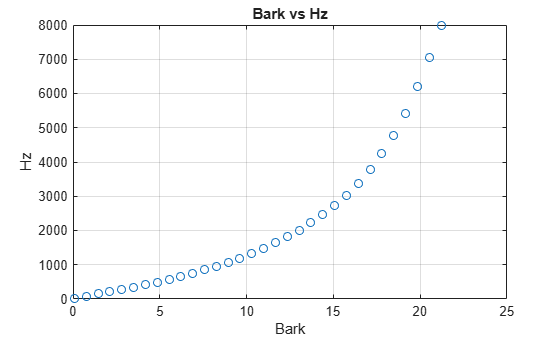# hz2bark

Convert from hertz to Bark scale

## Syntax

``bark = hz2erb(hz)``

## Description

example

````bark = hz2erb(hz)` converts values in hertz to values on the Bark frequency scale.```

## Examples

collapse all

Set two bounding frequencies in Hz and then convert them to the Bark scale.

`b = hz2bark([20,8000]);`

Generate a row vector of 32 values uniformly spaced on the Bark scale.

`barkVect = linspace(b(1),b(2),32);`

Convert the row vector of values into equivalent frequencies in Hz.

`hzVect = bark2hz(barkVect);`

Plot the two vectors for comparison. As Bark values increase linearly, Hz values increase exponentially.

```plot(barkVect,hzVect,'o') title('Bark vs Hz') xlabel('Bark') ylabel('Hz') grid on```## Input Arguments

collapse all

Input frequency in Hz, specified as a scalar, vector, matrix, or multidimensional array.

Data Types: `single` | `double`

## Output Arguments

collapse all

Output frequency on the Bark scale, returned as a scalar, vector, matrix, or multidimensional array the same size as `hz`.

Data Types: `single` | `double`

## Algorithms

The frequency conversion from Hz to the Bark scale uses the following formula:

`$\begin{array}{l}bark=\frac{\left(26.81\right)\left(hz\right)}{1960+hz}-0.53\\ if:bark<2\to bark=bark+\left(0.15\right)\left(2-bark\right)\\ if:bark>20.1\to bark=bark+\left(0.22\right)\left(bark-20.1\right)\end{array}$`

The Bark value correction occurs after the conversion from Hz to the Bark scale.

 Traunmüller, Hartmut. "Analytical Expressions for the Tonotopic Sensory Scale." Journal of the Acoustical Society of America. Vol. 88, Issue 1, 1990, pp. 97–100.

##### SupportGet trial now